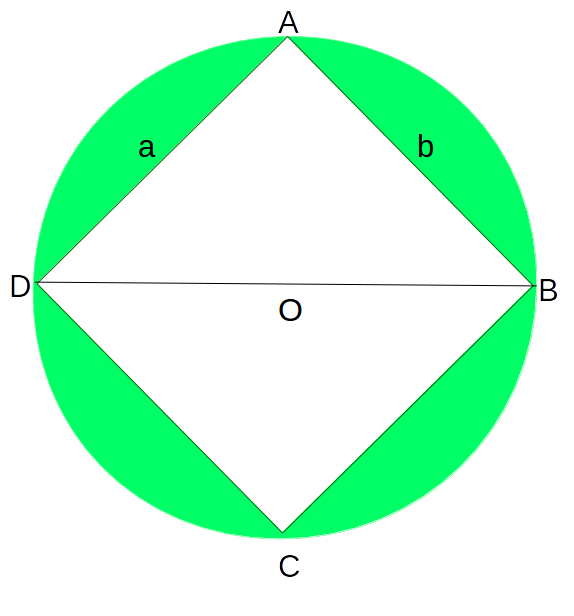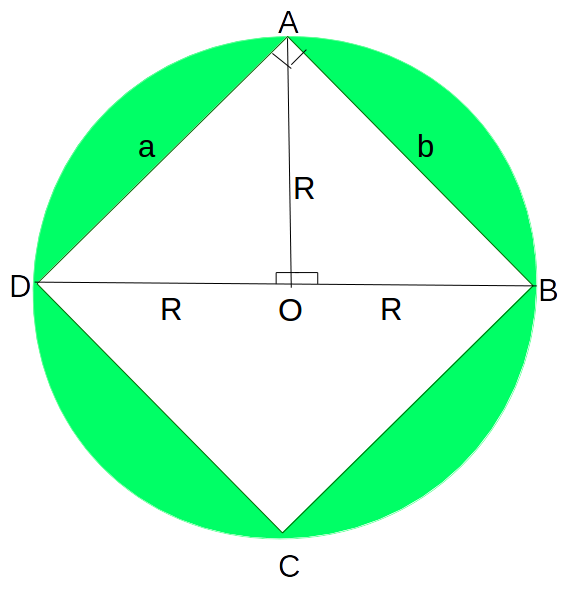# Number of rectangles in a circle of radius R

Given a circular sheet of radius R and the task is to find the total number of rectangles with integral length and width that can be cut from the circular sheet, one at a time.

Examples:

Input: R = 2
Output: 8
8 rectangles can be cut from a circular sheet of radius 2.
These are: 1×1, 1×2, 2×1, 2×2, 1×3, 3×1, 2×3, 3×2.

Input: R = 1
Output: 1
Only one rectangle with dimensions 1 X 1 is possible.

## Recommended: Please solve it on “PRACTICE ” first, before moving on to the solution

Approach
Consider the following diagram,Its easy to see, that ABCD is the largest rectangle that can be formed in the given circle with radius R and centre O, having dimensions a X b

Drop a perpendicular AO such that, ∠AOD = ∠AOB = 90°

Consider the following diagram for further analysis,```Consider triangles AOD and AOB,
In these triangles,
AO = AO (Common Side)
∠AOD = ∠AOB = 90°
OD = OB = R
Thus, by SAS congruence ▵AOD ≅ ▵AOB
∴ AD = AB by CPCT(i.e Corresponding Parts on Congruent Triangles)
or, a = b
=> The rectangle ABCD is a square
```

The diameter BD is the maximum diagonal the rectangle can have to be able to be cut from the Circular Sheet.

Thus, all the combinations of a and b can be checked to form all possible rectangles, and if the diagonal of any such rectangle is less than or equal to the length of the diagonal of the largest rectangle formed (i.e 2 * R, where R is the Radius of the circle as explained above)

Now, the maximum length of a and b will always be strictly less than the diameter of the circle so all possible values of a and b will lie in the closed interval [1, (2 * R – 1)].

Below is the implementation of the above approach:

## C++

 `// C++ program to find the number of rectangles ` `// that can be cut from a circle of Radius R ` `#include ` `using` `namespace` `std; ` ` `  `// Function to return the total possible ` `// rectangles that can be cut from the circle ` `int` `countRectangles(``int` `radius) ` `{ ` ` `  `    ``int` `rectangles = 0; ` ` `  `    ``// Diameter = 2 * Radius ` `    ``int` `diameter = 2 * radius; ` ` `  `    ``// Square of diameter which is the square ` `    ``// of the maximum length diagnal ` `    ``int` `diameterSquare = diameter * diameter; ` ` `  `    ``// generate all combinations of a and b ` `    ``// in the range (1, (2 * Radius - 1))(Both inclusive) ` `    ``for` `(``int` `a = 1; a < 2 * radius; a++) { ` `        ``for` `(``int` `b = 1; b < 2 * radius; b++) { ` ` `  `            ``// Calculate the Diagnal length of ` `            ``// this rectange ` `            ``int` `diagnalLengthSquare = (a * a + b * b); ` ` `  `            ``// If this rectangle's Diagonal Length ` `            ``// is less than the Diameter, it is a ` `            ``// valid rectangle, thus increment counter ` `            ``if` `(diagnalLengthSquare <= diameterSquare) { ` `                ``rectangles++; ` `            ``} ` `        ``} ` `    ``} ` ` `  `    ``return` `rectangles; ` `} ` ` `  `// Driver Code ` `int` `main() ` `{ ` ` `  `    ``// Radius of the circle ` `    ``int` `radius = 2; ` ` `  `    ``int` `totalRectangles; ` `    ``totalRectangles = countRectangles(radius); ` `    ``cout << totalRectangles << ``" rectangles can be"` `         ``<< ``"cut from a circle of Radius "` `<< radius; ` `    ``return` `0; ` `} `

## Java

 `// Java program to find the  ` `// number of rectangles that  ` `// can be cut from a circle ` `// of Radius R ` `import` `java.io.*; ` ` `  `class` `GFG ` `{ ` `     `  `// Function to return  ` `// the total possible ` `// rectangles that can  ` `// be cut from the circle ` `static` `int` `countRectangles(``int` `radius) ` `{ ` `    ``int` `rectangles = ``0``; ` ` `  `    ``// Diameter = 2 * Radius ` `    ``int` `diameter = ``2` `* radius; ` ` `  `    ``// Square of diameter  ` `    ``// which is the square ` `    ``// of the maximum length  ` `    ``// diagnal ` `    ``int` `diameterSquare = diameter * ` `                         ``diameter; ` ` `  `    ``// generate all combinations  ` `    ``// of a and b in the range  ` `    ``// (1, (2 * Radius - 1)) ` `    ``// (Both inclusive) ` `    ``for` `(``int` `a = ``1``;  ` `             ``a < ``2` `* radius; a++) ` `    ``{ ` `        ``for` `(``int` `b = ``1``; ` `                 ``b < ``2` `* radius; b++) ` `        ``{ ` ` `  `            ``// Calculate the  ` `            ``// Diagnal length of ` `            ``// this rectange ` `            ``int` `diagnalLengthSquare = (a * a +  ` `                                       ``b * b); ` ` `  `            ``// If this rectangle's Diagonal ` `            ``// Length is less than the Diameter,  ` `            ``// it is a valid rectangle, thus  ` `            ``// increment counter ` `            ``if` `(diagnalLengthSquare <= diameterSquare) ` `            ``{ ` `                ``rectangles++; ` `            ``} ` `        ``} ` `    ``} ` ` `  `    ``return` `rectangles; ` `} ` ` `  `// Driver Code ` `public` `static` `void` `main (String[] args)  ` `{ ` `     `  `// Radius of the circle ` `int` `radius = ``2``; ` ` `  `int` `totalRectangles; ` `totalRectangles = countRectangles(radius); ` `System.out.println(totalRectangles +  ` `             ``" rectangles can be "` `+  ` `            ``"cut from a circle of"` `+  ` `               ``" Radius "` `+ radius); ` `} ` `} ` ` `  `// This code is contributed ` `// by anuj_67. `

## Python3

 `# Python3 program to find  ` `# the number of rectangles ` `# that can be cut from a  ` `# circle of Radius R Function ` `# to return the total possible ` `# rectangles that can be cut ` `# from the circle ` `def` `countRectangles(radius): ` `     `  `    ``rectangles ``=` `0` `     `  `    ``# Diameter = 2 * Radius ` `    ``diameter ``=` `2` `*` `radius ` `     `  `    ``# Square of diameter which  ` `    ``# is the square of the  ` `    ``# maximum length diagnal ` `    ``diameterSquare ``=` `diameter ``*` `diameter ` `     `  `    ``# generate all combinations  ` `    ``# of a and b in the range  ` `    ``# (1, (2 * Radius - 1))(Both inclusive) ` `    ``for` `a ``in` `range``(``1``, ``2` `*` `radius):  ` `        ``for` `b ``in` `range``(``1``, ``2` `*` `radius): ` `             `  `            ``# Calculate the Diagnal  ` `            ``# length of this rectange ` `            ``diagnalLengthSquare ``=` `(a ``*` `a ``+`  `                                   ``b ``*` `b) ` `             `  `            ``# If this rectangle's Diagonal  ` `            ``# Length is less than the  ` `            ``# Diameter, it is a valid ` `            ``# rectangle, thus increment counter ` `            ``if` `(diagnalLengthSquare <``=` `diameterSquare) : ` `                ``rectangles ``+``=` `1` `     `  `    ``return` `rectangles ` ` `  `# Driver Code ` ` `  `# Radius of the circle ` `radius ``=` `2` `totalRectangles ``=` `countRectangles(radius) ` `print``(totalRectangles , ``"rectangles can be"` `,  ` `      ``"cut from a circle of Radius"` `, radius) ` ` `  `# This code is contributed by Smita `

## C#

 `// C# program to find the  ` `// number of rectangles that  ` `// can be cut from a circle ` `// of Radius R ` `using` `System; ` ` `  `class` `GFG ` `{ ` `     `  `// Function to return  ` `// the total possible ` `// rectangles that can  ` `// be cut from the circle ` `static` `int` `countRectangles(``int` `radius) ` `{ ` `    ``int` `rectangles = 0; ` ` `  `    ``// Diameter = 2 * Radius ` `    ``int` `diameter = 2 * radius; ` ` `  `    ``// Square of diameter  ` `    ``// which is the square ` `    ``// of the maximum length  ` `    ``// diagnal ` `    ``int` `diameterSquare = diameter * ` `                         ``diameter; ` ` `  `    ``// generate all combinations  ` `    ``// of a and b in the range  ` `    ``// (1, (2 * Radius - 1)) ` `    ``// (Both inclusive) ` `    ``for` `(``int` `a = 1;  ` `             ``a < 2 * radius; a++) ` `    ``{ ` `        ``for` `(``int` `b = 1; ` `                 ``b < 2 * radius; b++) ` `        ``{ ` ` `  `            ``// Calculate the  ` `            ``// Diagnal length of ` `            ``// this rectange ` `            ``int` `diagnalLengthSquare = (a * a +  ` `                                        ``b * b); ` ` `  `            ``// If this rectangle's  ` `            ``// Diagonal Length is  ` `            ``// less than the Diameter,  ` `            ``// it is a valid rectangle,  ` `            ``// thus increment counter ` `            ``if` `(diagnalLengthSquare <=  ` `                             ``diameterSquare) ` `            ``{ ` `                ``rectangles++; ` `            ``} ` `        ``} ` `    ``} ` ` `  `    ``return` `rectangles; ` `} ` ` `  `// Driver Code ` `public` `static` `void` `Main ()  ` `{ ` `     `  `// Radius of the circle ` `int` `radius = 2; ` ` `  `int` `totalRectangles; ` `totalRectangles = countRectangles(radius); ` `Console.WriteLine(totalRectangles +  ` `            ``" rectangles can be "` `+  ` `           ``"cut from a circle of"` `+  ` `              ``" Radius "` `+ radius); ` `} ` `} ` ` `  `// This code is contributed ` `// by anuj_67. `

## PHP

 ` `

Output:

```8 rectangles can be cut from a circle of Radius 2
```

Time Complexity: O(R2), where R is the Radius of the Circle

GeeksforGeeks has prepared a complete interview preparation course with premium videos, theory, practice problems, TA support and many more features. Please refer Placement 100 for details

My Personal Notes arrow_drop_upCheck out this Author's contributed articles.

If you like GeeksforGeeks and would like to contribute, you can also write an article using contribute.geeksforgeeks.org or mail your article to contribute@geeksforgeeks.org. See your article appearing on the GeeksforGeeks main page and help other Geeks.

Please Improve this article if you find anything incorrect by clicking on the "Improve Article" button below.

Improved By : vt_m, Smitha Dinesh Semwal

Article Tags :
Practice Tags :

1

Please write to us at contribute@geeksforgeeks.org to report any issue with the above content.